# Dimension reduction

Modified on Wed, 13 Sep 2023 at 02:30 PM

# Introduction

Dimension reduction is a way to reduce your high-dimensional data to a lower dimension (e.g., from 20000 features to 2 components), to make the analysis and visualization of the data easier. The objective is to remove data that is highly redundant, while maintaining the maximum amount of information (hence minimizing the loss of information). How information is defined depends on the dimension reduction algorithm itself. The dimension reduction step is now a staple in biological data analysis due to the high amount of features measured in the omics field.

The UniApp enables you to perform dimension reduction on all type of datasets, visualize the results as a 2D plot and customize such plot as needed: you can color code the plot by using metadata variables, the original features expression/abundance or the engineered features. The dimension reduction methods available are principal component analysis (PCA), t-distributed stochastic neighbor embedding (t-SNE) and uniform manifold approximation and projection (UMAP).

# 1. Creating a plot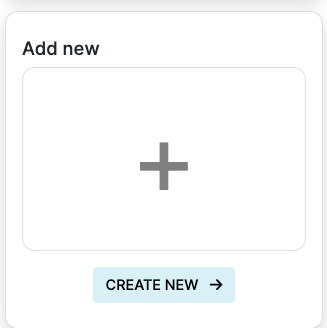As a first step of the analysis, a plot must be created by clicking on the create plot icon in your analysis track. This will lead to a section where the analysis of interest can be selected.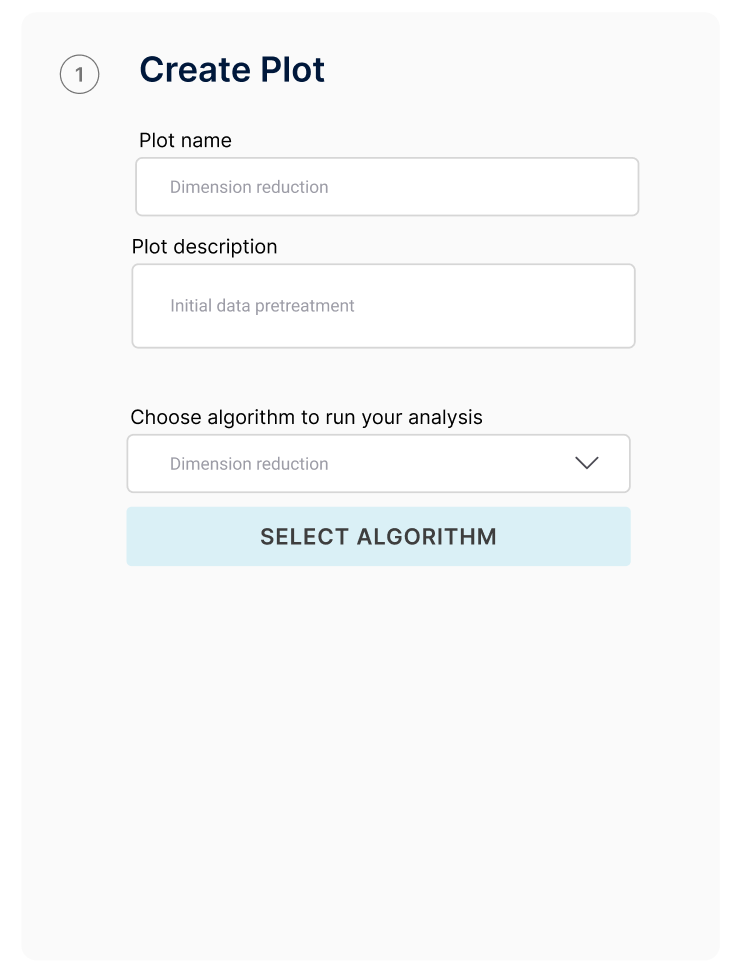In order to ensure efficient organization, a name and description must be assigned to the analysis under the appropriate fields. Subsequently under "Choose algorithm to run your analysis" Dimension reduction must be selected.

#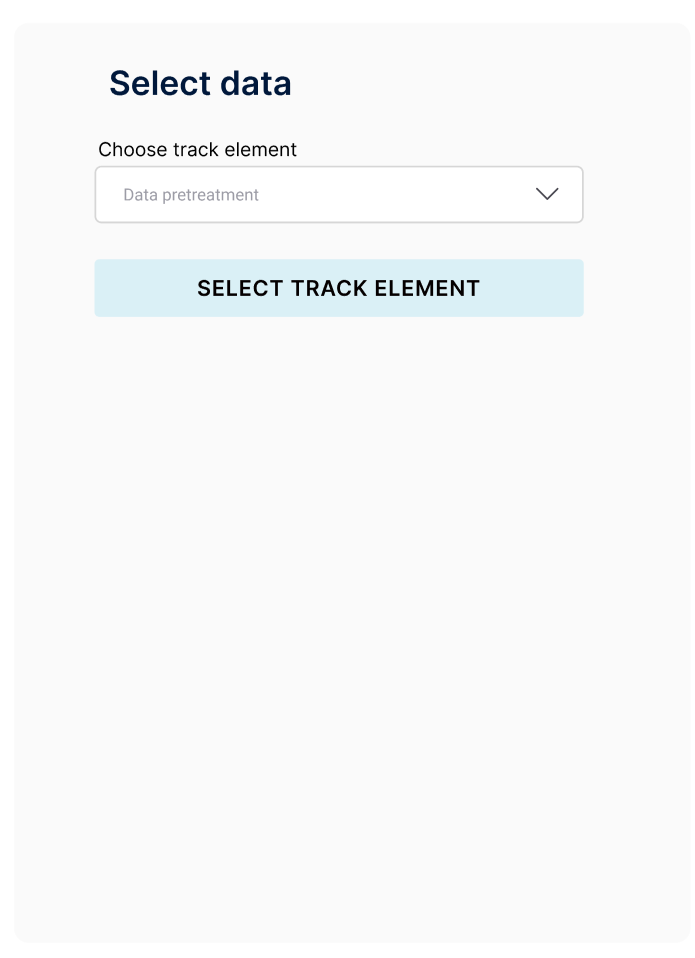In the field "Choose track element", the input data can be selected. To confirm your selection click on the Select track element button.

# 3. Setting parameters

The pivot table has not parameters to select so you can proceed to run you analysis by click the

##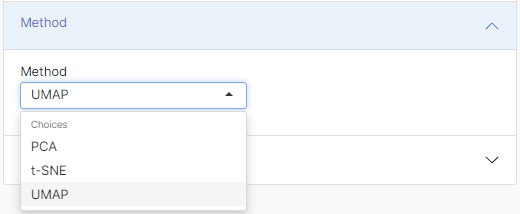Principal components analysis (PCA) is one of the most commonly used dimension reduction technique, which is routinely used for the data exploration analysis and visualization of high dimensional data. PCA is a statistical procedure based on the eigenvalue decomposition method and has been used across many fields of research, including biological sciences. PCA first captures the differences between all variables, and then identifies new variables (components) as the linear combinations of the original variables. These variables are known as principal components and each of them represents a specific characteristic defined by the associated variable. The first principal component explains the maximum amount of variance in the data, and the next component explains the second most amount of variance in the data, as so on. By using the first two principal components, all samples in the data can easily be visualized in a 2D plot, which can reveal the underlying structure of the high dimensional data.

t-distributed stochastic neighbor embedding (t-SNE) is a nonlinear dimension reduction technique. t-SNE is suitable for the visualization of high dimensional data in low dimension (two or three-dimensional) space. t-SNE captures the non-linear structure of the data by using the local relationships between the data points and creates a mapping onto a low-dimensional space. The relationship between the data points in the high dimensional space is defined by this Gaussian based distribution, then a Student t-distribution is used for the recreating a similar probability distribution in the low dimensional space. With respect to other commonly used dimension reduction techniques (e.g. PCA), t-SNE has the advantage of finding non-linear relationships in the data, maintaining the high dimensional structure of the data in the low dimensional mapping. Therefore t-SNE is suitable for different types of datasets, for example single cell RNA-seq data. t-SNE is a non-convex, non-deterministic approach, meaning it can have many local minima: t-SNE can give different results based on the selected parameters because there are no failsafe methods to reach the global minima.

Uniform manifold approximation and projection (UMAP) is a nonlinear dimension reduction technique. It is similar to t-SNE, but it can preserve the global structure in a more efficient way. It tries to strike the balance between preserving the global and local structure of the data.

It is important to note that while with PCA you can select to compute an arbitrary number of components, and then plot just the first two components with no problems, this will not be correct with t-SNE and UMAP. t-SNE and UMAP create a low-dimensional mapping in the dimension you specify: mapping the same data to a two dimensional space or to a three dimensional space will generate completely different mappings. In short, if you want to visualize the t-SNE or UMAP in two dimensions, the Dimensions parameter must be set to 2.

## 3.2 Dimension reduction method settings

When you click on Parameter tab, you can start to define the parameters for the dimension reduction method you select. The parameters will change based on the dimension reduction method you selected.

###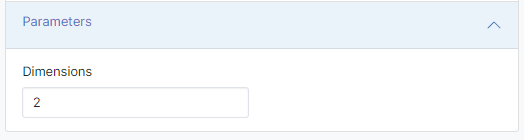The parameters you can set are the following:

• Dimensions: how many dimensions/components you want to compute.

###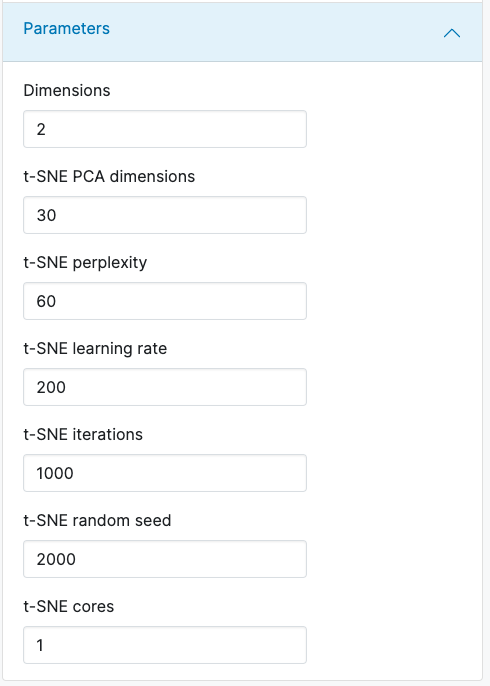The parameters you can set are the following:

• Dimensions: how many dimensions/components you want to compute.
• Number of PCA dimensions: before computing the t-SNE reduction, it is advisable to perform a PCA reduction, which will then be fed to the t-SNE algorithm. This is due to the fact that computing t-SNE on all the features on big datasets is unfeasible (time-wise). This parameter indicates how many PCA dimensions you want to use when calculating the t-SNE reduction. If you want to calculate the t-SNE reduction on the full data (not advisable for big datasets), you can put the value to 0.
• Perplexity: it indicates (loosely) how to balance the attention between the local and global aspects of your data. The parameter is a guess about the number of how many close neighbors each point has. Larger perplexities will take more global structure into account, whereas smaller perplexities will make the embeddings more locally focused. The higher the perplexity, the more intensive the computation will become.
• Learning rate: if the learning rate is too high, the data may look like a ‘ball’ with any point approximately equidistant from its nearest neighbours. If the learning rate is too low, most points may look compressed in a dense cloud with few outliers. If the cost function gets stuck in a bad local minimum increasing the learning rate may help.
• Iterations: maximum number of iterations for the optimization. If you see a t-SNE plot with strange “pinched” shapes, chances are the process was stopped too early. There is no fixed number of steps that yields a stable result: different data sets can require different numbers of iterations to converge.
• Random seed: since t-SNE is a stochastic algorithm, the same random seed must be used to reproduce the same results when using the same parameters.
• Cores: the number of cores to use during the computation. It is important to note that when using multiple cores, the computed results will be slightly different across runs (even when using the same parameters, random seed included).
As usual, the default parameters provided by the UniApp can be a good starting point to analyse the data, but these parameters must be optimized individually for each dataset: there is no rule that says that you need to use a Perplexity of 100 instead of 200. Try to experiment with different parameters.

### 3.2.3 UMAP parameters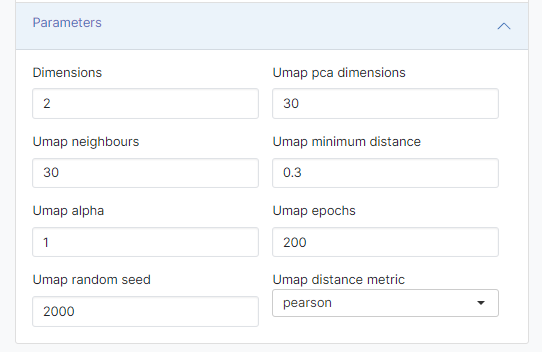The parameters you can set are the following:

• Dimensions: how many dimensions/components you want to compute
• Number of PCA dimensions: before computing the UMAP reduction, it is advisable to perform a PCA reduction, which will then be fed to the UMAP algorithm. This is due to the fact that computing UMAP on all the features on big datasets is unfeasible (time-wise). This parameter indicates how many PCA dimensions you want to use when calculating the UMAP reduction. If you want to calculate the UMAP reduction on the full data (not advisable for big datasets), you can put the value to 0.
• Number of nearest neighbours: it determines the number of neighboring points used in the local approximations of the manifold structure. Larger values will result in more global structure being preserved (at the loss of detail in the local structure).
• Minimum distance: this controls how tightly the embedding is allowed compress points together. Larger values ensure embedded points to be more evenly distributed, while smaller values allow the algorithm to optimise more accurately with regard to the local structure. Sensible values are in the range 0.001-0.5.
• Alpha: The initial learning rate for UMAP optimization.
• Epochs: Use this option to specify the number of training epochs to be used in optimizing the low dimensional embedding. Larger values result in more accurate embeddings. 200 epochs are specified by default. At some point increasing the number of epochs wouldn’t yield enough improved results to justify the longer processing times.
• Random seed: since UMAP is a stochastic algorithm, the same random seed must be used to reproduce the same results when using the same parameters.
• Distance metric: this controls how distance is computed in the ambient space of the input data.
As usual, the default parameters provided by the UniApp can be a good starting point to analyse the data, but these parameters must be optimized individually for each dataset: there is no rule that says that you need to use a Number of nearest neighbours of 30 instead of 15. Try to experiment with different parameters!

#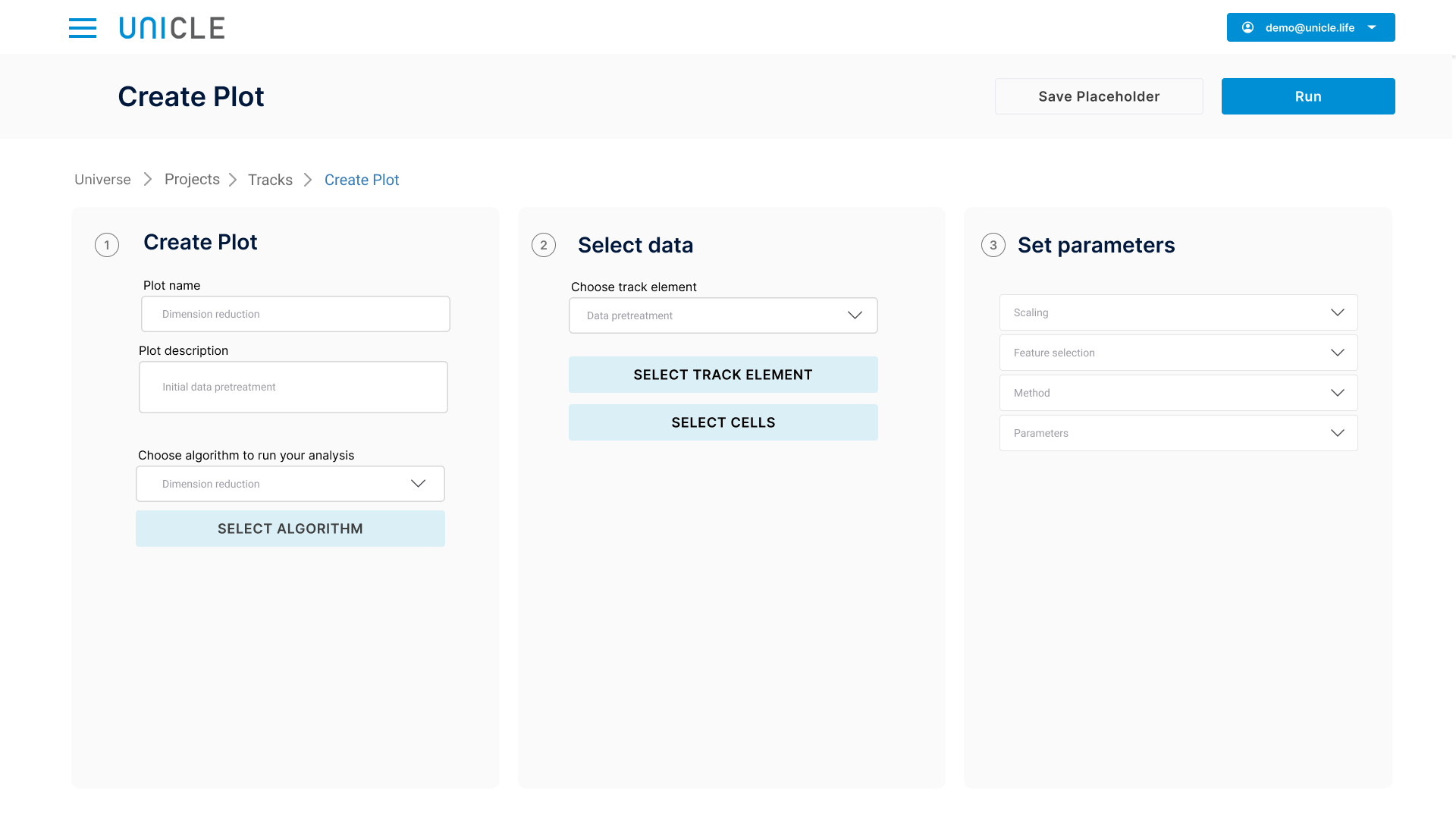When the parameters are all set-up, you can click on the Run button to compute the dimension reduction results. This could take quite some time, depending on the dimension reduction method (t-SNE is much more intensive than PCA) and the size of the data (it could take several hours for extremely big datasets).

As soon as the reduction is computed, a plot will appear, where each dot in the plot is an observation of your data. Usually, the more these dots are far apart, the more dissimilar those observations will be.

#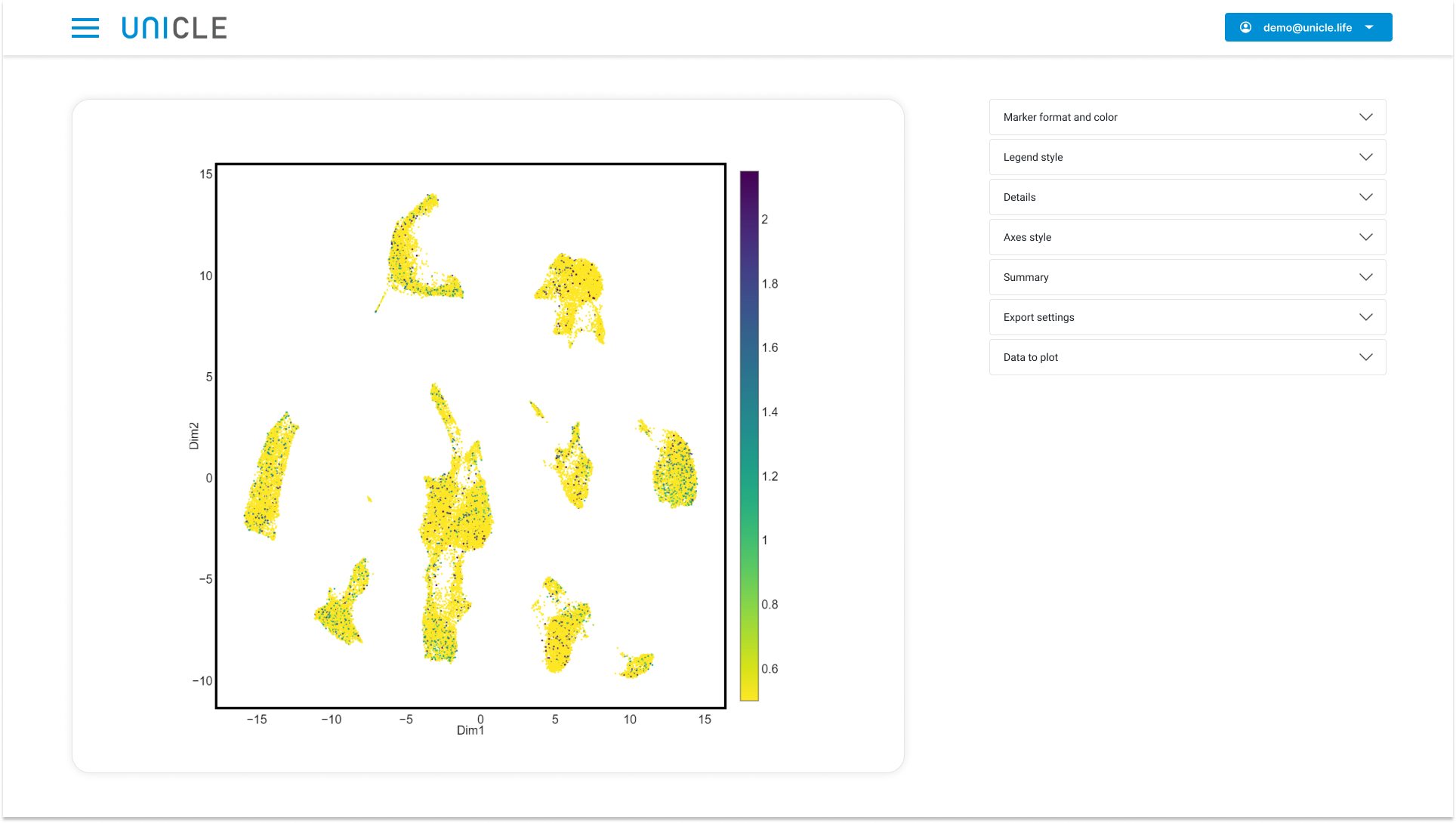## 5.1 Color coding

Once the dimension reduction result is available, you can color code the dimension reduction plot by any feature or variable you want. To color code the plot by a metadata variable, you need to go to >Select input >Color coding >Metadata , and then select the variable you want to visualize. under 'Colour by'.

To color code the plot by a feature (e.g. a gene), you need to select a data matrix with original features, and then select the feature of interest.

If you want to color code the plot by an engineered feature (e.g. a pathway), you need to select a data matrix with engineered features (created with Feature engineering module), and then select the engineered feature of interest.

All dropdown boxes in BIOMEX are searchable, so you can search for the variable/feature of interest by typing it out. This way you do not have to scroll down to find your variable/feature of interest.

## 5.2 Marker format and color

In the Marker format and color tab you can customize the marker that appears on the dimension reduction plot. The marker customization options vary slightly depending of type of variable visualized.

### 5.2.1 Marker format and color for categorical variables

• Individual or shared markers: for categorical variables only. This option decides if you will share the same customization options for all categories or if you will set specific customization options for each category.
• Marker symbol: change marker symbol.
• Marker size: adjust marker size.
• Marker opacity: adjust marker opacity.
• Metadata color scheme: choose different color scheme.
• Maximum of categories to plot: prevents rendering of plot with too many categories.

• ### Marker symbol: change marker symbol.

• Marker size: adjust marker size.
• Marker opacity: adjust marker opacity.
• Gene expression color scale: choose different color scheme.
• Color scale gradient: adjust color scale. Increasing this values is useful when only plotting cells with high expression of a certain gene.
• Reverse color scale: reverses color scale.

## 5.3 Legend style

Customizes plot legend or scale bar.

• Show/hide legend: toggle to display legend.
• Show/hide scale:  toggle to display/hide scaling.
• Legend title: add legend title.
• Font size of the legend title: changes legend title font size.
• Legend position x-direction: changes legend position on the x axis.
• Legend position y-direction: changes legend position on the y axis.
• Font size of the legend: changes font size of the legend.

## 5.4 Details

### 5.4.1 Grid style

• Show gridtoggles grid.
• Grid color: changes grid color.
• Border width: changes width of plot border.

• ### Title: sets plot title.

• Title font sizeadjusts plot title font size.
• Legend position x-directionchanges plot title position on the x axis.
• Legend position y-direction: changes plot title position on the y axis.

• ### Margin bottom: sets bottom margin.

• Margin left: sets left margin.
• Margin right: sets right margin.
• Margin top: sets top margin.

### 5.4.4 Font style

• Font size: sets font size.
• Font type: sets font type.
• Font: sets font color.

## 5.5 Axes style

Here you can edit the axis style for the x,y and z axes.

• Axis labelsets axis label.
• Invert axis: inverts axis.
• Dimension to plot on axis: set dimension to plot on axis. In PCA you can generate plot from different principle components using this option.

## 5.6 Summary

### 5.6.1 Subsampling and summarization

Visualizing extremely large single cell data sets can be problematic. With subsampling and summarization you can plot a sumarized representation of your data.

• Maximum number of markers to show: determines maximum number of markers that will be displayed.
• Resolution of the summarization grid: sets resolution of the grid.
• Method of data summarization: selects method of data summarization.

In development.

## 5.7 Export settings

Here you can prepare your plot for export.

• Export formatsets plot file format.
• Width of plot: adjusts plot width.
• Height of plot: adjusts plot height.
• File name: set file name for exported plot.

## 5.8 Data to plot

Toggles display of dimension reduction plot or elbow plot. An elbow plot displays the data's principle components arranged by percent of variance explained in decreasing order. The elbow plot is useful when deciding the number of PCs to input to t-SNE or UMAP.